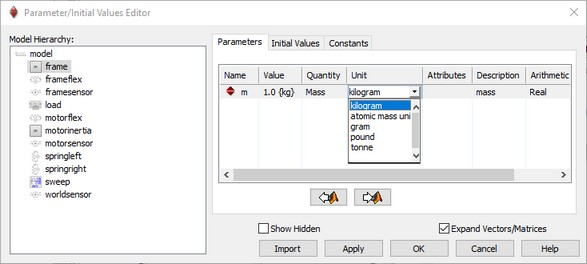﻿ 20-sim webhelp > Editor > Domains, Quantities and Units > Quantities and Units

Quantities and Units

In 20-sim, to every constant, parameter and variable a quantity may be assigned. Well known quantities are for example position, velocity, time and energy. 20-sim will use these quantities to check the validity of (basic) equations. The value of a quantity can be expressed in a specific unit. The quantity time can for example be expressed in the unit second or in the unit minute:

 quantity unit multiplication to SI unit Time second (SI unit) minute hour day year 1 60 3600 86400 31556926 Position meter (SI unit) foot inch yard 1 0.3048 0.0254 0.9144

In 20-sim a quantity can be expressed in every desired unit. To prevent conversion problems, internal calculations are always performed in standard SI-units. Therefore non-SI units are stored with a multiplication factor. When a variable has a unit, the unit can also have common engineering prefixes. For example, a parameter m {kg} with a value of 0.1 can be selected in the Parameters / Initial Values Editor. If you click on the unit, you can select other units to enter the mass.You can use the units in the Parameters / Initial Values Editor to select a desired unit.

You can enter new quantities and units in the Domains, Quantities and Units Editor.